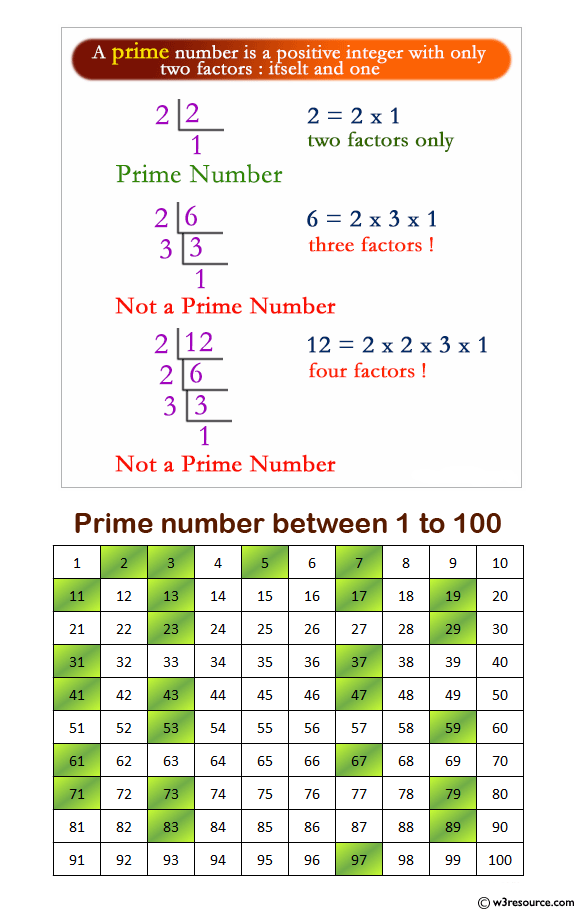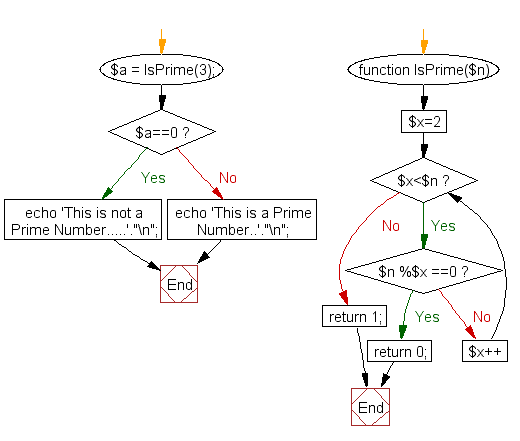﻿ PHP Function Exercise: Check whether a number is prime or not - w3resource# PHP function Exercises: Check whether a number is prime or not

## PHP function: Exercise-2 with Solution

Write a function to check whether a number is prime or not.

Note: A prime number (or a prime) is a natural number greater than 1 that has no positive divisors other than 1 and itself.

Pictorial Presentation:Sample Solution:

PHP Code:

``````<?php
function IsPrime(\$n)
{
for(\$x=2; \$x<\$n; \$x++)
{
if(\$n %\$x ==0)
{
return 0;
}
}
return 1;
}
\$a = IsPrime(3);
if (\$a==0)
echo 'This is not a Prime Number.....'."\n";
else
echo 'This is a Prime Number..'."\n";
?>
```
```

Sample Output:

```This is a Prime Number..
```

Flowchart :PHP Code Editor:

Have another way to solve this solution? Contribute your code (and comments) through Disqus.

What is the difficulty level of this exercise?

Test your Programming skills with w3resource's quiz.

﻿

## PHP: Tips of the Day

Formatting a number with leading zeros in PHP

Use sprintf :

`sprintf('%08d', 1234567);`

Alternatively you can also use str_pad:

`str_pad(\$value, 8, '0', STR_PAD_LEFT);`

Ref : https://bit.ly/3fS2OLd